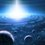# Square root of i?

What is wrong with this proof or is it in fact it is valid?

$\large \sqrt{i} = (i)^{\frac{1}{2}} = (i)^{\frac{4}{8}} = \sqrt{i^{4}} = \sqrt{1} =$

$1, -1, i, -i, \sqrt{i}, -\sqrt{i} \sqrt{-i} \text{and} -\sqrt{-i}.$

Does that mean that all 8 solutions are solutions of $\sqrt{i}$?

I know that $\sqrt{i}$ can be expressed as $\pm\frac{1}{\sqrt{2}}\times(1+i)$ but my questions are:

1) Which of the 8 solutions are invalid and why are they invalid?

2) Is it possible of $\sqrt{i}$ to have more than 2 solutions? If so, what are they?

3) Is it possible for $\sqrt{i}$ to have any solutions where there is a real part (in addition to the 2 given)?Note by Eamon Gupta
5 years, 12 months ago

This discussion board is a place to discuss our Daily Challenges and the math and science related to those challenges. Explanations are more than just a solution — they should explain the steps and thinking strategies that you used to obtain the solution. Comments should further the discussion of math and science.

When posting on Brilliant:

• Use the emojis to react to an explanation, whether you're congratulating a job well done , or just really confused .
• Ask specific questions about the challenge or the steps in somebody's explanation. Well-posed questions can add a lot to the discussion, but posting "I don't understand!" doesn't help anyone.
• Try to contribute something new to the discussion, whether it is an extension, generalization or other idea related to the challenge.

MarkdownAppears as
*italics* or _italics_ italics
**bold** or __bold__ bold
- bulleted- list
• bulleted
• list
1. numbered2. list
1. numbered
2. list
Note: you must add a full line of space before and after lists for them to show up correctly
paragraph 1paragraph 2

paragraph 1

paragraph 2

[example link](https://brilliant.org)example link
> This is a quote
This is a quote
    # I indented these lines
# 4 spaces, and now they show
# up as a code block.

print "hello world"
# I indented these lines
# 4 spaces, and now they show
# up as a code block.

print "hello world"
MathAppears as
Remember to wrap math in $$ ... $$ or $ ... $ to ensure proper formatting.
2 \times 3 $2 \times 3$
2^{34} $2^{34}$
a_{i-1} $a_{i-1}$
\frac{2}{3} $\frac{2}{3}$
\sqrt{2} $\sqrt{2}$
\sum_{i=1}^3 $\sum_{i=1}^3$
\sin \theta $\sin \theta$
\boxed{123} $\boxed{123}$

Sort by:

Finding the square roots of $i$ is the same thing as solving the equation $x^2=i$ in complex numbers.

Now, what you did is raise both sides of this equation to the fourth power to get $x^8=1$. While it is true that if $x^2=i$, then $x^8=1$, but the converse is not always true. In other words, when you are raising the equation to a power, you're introducing extraneous solutions.

Take this for example. If $x=4$, then $x^2=16$. But $x^2=16$ implies $x$ is equal to either $4$ or $-4$. So squaring gave you something ($x=-4$) that's not a solution to the orginal equation.

The fundamental theorem of algebra ensures that an $n$-degree polynomial with complex coefficients can have at most $n$ complex roots. So that means a complex number can not have more than two complex square roots. But higher dimensional numbers (like quaternions) are a different story.

- 5 years, 11 months ago

Ok thanks for clearing that up. I'll look into some quaternions...

- 5 years, 11 months ago

As $x=4$ and $x^2=16$ aren't same, $x^2=i$ and $\sqrt{i}$ aren't same too.

Solving $x^2=i$, we get $x=e^{\frac{i\pi} {4}}=\sqrt{-1},e^{-\frac{3i\pi}{4}}$.

But $\sqrt{i}$ means principal root of $i$ which is $\sqrt{-1}$

- 5 years, 11 months ago

It is possible to extend the definition of a principle square root to an arbitrary complex number, but it's usually not done because it is not as useful as the principal square root of a non-negative real. (The definition you're using is something like this: the principal square root is the one with the positive real part or the one that lies in the right-complex-half-plane, or $\sqrt{z}=\sqrt{|z|}\frac{z+|z|}{|z+|z||}$) That's why the principal square root operator, $\sqrt{\quad}$ is generally not used on a general complex number. It doesn't offer us much to talk about a particular square root of a general complex number.

The OP was actually looking for the square roots (plural) of $e^{i\frac{\pi}{2}}$ [even though they didn't state it explicitly] and I was aiming to address that.

- 5 years, 11 months ago

- 5 years, 11 months ago

It can be use in hard forms

- 5 years, 11 months ago